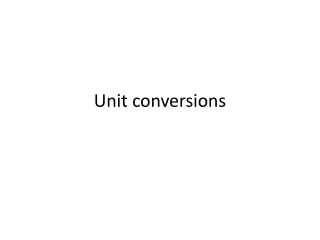DownloadDownload PresentationUnit conversions

# Unit conversions

Télécharger la présentation## Unit conversions

- - - - - - - - - - - - - - - - - - - - - - - - - - - E N D - - - - - - - - - - - - - - - - - - - - - - - - - - -
##### Presentation Transcript

1. Unit conversions

2. Stochastic versus ODE ODE Stochastic

3. Units • With concentrations* (ODE simulations only): • concentrations (eg moles/liter) for species • Unit concentration / unit time for reactions • With particles (ODE or stochastic): • Number of molecules for species • Reaction firings per unit time * Usual way that measurements are reported in the literature.

4. Concentrations<->Particles • NA = Avogadro’s Number, V=volume • Concentration C -> C*NA*V particles • Particles P -> P/(NA*V) concentration (eg moles/liter)

5. Mass Action • A->C, rate k1: • [C] = k1[A]: Units of k1 must be /time • A+B->C, rate k2: • [C] = k2[A][B]: Units of k2 must be /conc/time • ->C, rate k0: • [C] = k0: Units of k0 must be conc/time

6. Unimolecular reactionsA -> B Concentrations Particles A->B k1’ Units: /time • A->B k1 • Units: /time Conversion: k1’ = k1

7. Avogadro’s constant • The Avogadro constant (NA) is defined as the ratio of the number of constituent particles N (usually atoms or molecules) in a sample to the amount of substance n (unit mole) through the relationship • NA = N/n • Wikipedia • NA = 6.02214129×1023 mol−1

8. Bimolecular reactions Concentrations Particles A+B -> C k2’ Units: /time • A+B -> C k2 • Units: /conc/time Conversion: k2’ = k2/(NA*V)

9. Constant Reaction Concentrations Particles -> C k0’ Units: /time • -> C k0 • Units: conc/time Conversion: k0’ = k0*(NA*V)

10. Review: MichaelisMenten

11. Special Feature • explicit enzyme:: S + E -> P + E Sat(kcat,Km) • Implicit enzyme(!): S -> P Sat(Vmax,Km)

12. Use the second method if… a) the enzyme is unknown b) the enzyme concentration is large and constant, and the user intends to run network-free simulations with NFsim.

13. Enzymatic Conversions • kcat' = kcat • Vmax' = Vmax*NA*V • Km' = Km*NA*V

14. Also… explicit enzyme: S + E -> P + E kcat/(Km + Stot) implicit enzyme: S -> P Vmax/(Km + Stot) Stot is an observable, giving the amount (concentration or #particles) of S Multiply reactant quantities times the formula